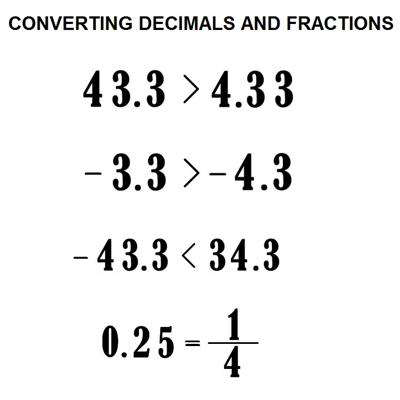Comparing Decimals and Fractions

Comparing decimals is very easy, but also very important. In fact, it’s the first thing we should learn to do in order to use real numbers (or decimal numbers) properly. A decimal number and a fractional number can be compared. One number is either greater than, less than or equal to the other number. When comparing fractional numbers to decimal numbers, convert the fraction to a decimal number by division and compare the decimal numbers.

It is easier to compare decimals when you have the same number of decimal digits. Thus, we often write extra zeros to the right of the last digit of one of the decimals being compared. These extra zeros are place holders and do not change the value of the decimal. However, if you inserted a zero between the decimal point and a decimal digit, that would change the value of the decimal.

To compare decimal numbers, start with tenths and then hundredths etc. If one decimal has a higher number in the tenths place then it is larger and the decimal with less tenths is smaller. If the tenths are equal compare the hundredths, then the thousandths etc. until one decimal is larger or there are no more places to compare.Key Concepts:

• Decimal notation is based on denominators that are powers of 10.
• Because we can write fractions with a denominator of 10 as an equivalent fraction with a denominator of 100, we can also write these numbers in decimal notation.
• Because decimal fractions can be written in either fraction or decimal form, the place value system holds when comparing decimals.

Fractions and decimals are examples of rational numbers. A rational number is any number that can be expressed as a ratio. Integers are rational numbers, but so are many of the numbers between them. Fractions and decimals are examples of rational numbers. A rational number is any number that can be expressed as a ratio. Integers are rational numbers, but so are many of the numbers between them.

It is often easy to estimate the decimal from a fraction. If this estimated decimal is obviously much larger or smaller than the compared decimal then it is not necessary to precisely convert the fraction to a decimal.

For example, let’s compare these two numbers: 343.45667 and 343.45567

We go through the digits one by one, and see that the first five digits are the same. But the sixth digit of the number 343.45667 is greater than the sixth digit in number 343.45567, which means that A is greater than B. Now, let’s do a couple more to really get the hang of this

information Source: